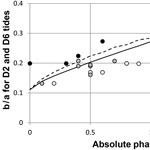Ocean Sci., 13, 599–607, 2017
https://doi.org/10.5194/os-13-599-2017
Ocean Sci., 13, 599–607, 2017
https://doi.org/10.5194/os-13-599-2017

Research article 20 Jul 2017

Research article | 20 Jul 2017# The double high tide at Port Ellen: Doodson's criterion revisited

Hannah A. M. Byrne, J. A. Mattias Green, and David G. Bowers Hannah A. M. Byrne et al.
• Bangor University, School of Ocean Sciences, Menai Bridge, Anglesey, LL59 5AB, UK

Abstract. Doodson proposed a minimum criterion to predict the occurrence of double high (or double low) waters when a higher-frequency tidal harmonic is added to the semi-diurnal tide. If the phasing of the harmonic is optimal, the condition for a double high water can be written bn2a > 1 where b is the amplitude of the higher harmonic, a is the amplitude of the semi-diurnal tide, and n is the ratio of their frequencies. Here we expand this criterion to allow for (i) a phase difference ϕ between the semi-diurnal tide and the harmonic and (ii) the fact that the double high water will disappear in the event that ba becomes large enough for the higher harmonic to be the dominant component of the tide. This can happen, for example, at places or times where the semi-diurnal tide is very small. The revised parameter is br2a, where r is a number generally less than n, although equal to n when ϕ = 0. The theory predicts that a double high tide will form when this parameter exceeds 1 and then disappear when it exceeds a value of order n2 and the higher harmonic becomes dominant. We test these predictions against observations at Port Ellen in the Inner Hebrides of Scotland. For most of the data set, the largest harmonic of the semi-diurnal tide is the sixth diurnal component, for which n = 3. The principal lunar and solar semi-diurnal tides are about equal at Port Ellen and so the semi-diurnal tide becomes very small twice a month at neap tides (here defined as the smallest fortnightly tidal range). A double high water forms when br2a first exceeds a minimum value of about 1.5 as neap tides are approached and then disappears as br2a then exceeds a second limiting value of about 10 at neap tides in agreement with the revised criterion.

Share